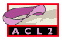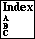THEORY-FUNCTIONS

functions for obtaining or producing theories
```Major Section:  THEORIES
```

```Example Calls of Theory Functions:
(universal-theory :here)
(union-theories th1 th2)
(set-difference-theories th1 th2)
```
The theory functions are documented individually:

• UNIVERSAL-THEORY -- all rules as of logical name

The functions (actually, macros) mentioned above are convenient ways to produce theories. (See theories.) Some, like `universal-theory`, take a logical name (see logical-name) as an argument and return the relevant theory as of the time that name was introduced. Others, like `union-theories`, take two theories and produce a new one. See redundant-events for a caution about the use of logical names in theory expressions.

Theory expressions are generally composed of applications of theory functions. Formally, theory expressions are expressions that involve, at most, the free variable `world` and that when evaluated with `world` bound to the current ACL2 world (see world) return theories. The ``theory functions'' are actually macros that expand into forms that involve the free variable `world`. Thus, for example `(universal-theory :here)` actually expands to `(universal-theory-fn :here world)` and when that form is evaluated with `world` bound to the current ACL2 world, `universal-theory-fn` scans the ACL2 property lists and computes the current universal theory. Because the theory functions all implicitly use `world`, the variable does not generally appear in anything the user types.